# verifying_clt_in_regression

## Verifying Central Limit Theorem in regression¶¶

In :
import pandas as pd
import numpy as np
import matplotlib.pyplot as plt
import seaborn as sns
%matplotlib inline
import warnings
warnings.filterwarnings('ignore')


### Synthesize the dataset¶¶

Create 1000 random integers between 0, 100 for X and create y such that $$y = \beta_{0} + \beta_{1}X + \epsilon$$ where $$\beta_{0} = 30 \ and \ \beta_{1} = 1.8 \ and \ \epsilon \ = \ standard \ normal \ error$$

In :
rand_1kx = np.random.randint(0,100,1000)
x_mean = np.mean(rand_1kx)
x_sd = np.std(rand_1kx)
x_mean

Out:
49.954
In :
pop_intercept = 30
pop_slope = 1.8
error_boost = 10
pop_error = np.random.standard_normal(size = rand_1kx.size) * error_boost
# I added an error booster since without it, the correlation was too high.

y = pop_intercept + pop_slope*rand_1kx + pop_error
y_mean = np.mean(y)
y_sd = np.std(y)
y_mean

Out:
119.4183378140413

Make a scatter plot of X and y variables.

In :
sns.jointplot(rand_1kx, y)

Out:
<seaborn.axisgrid.JointGrid at 0x1a1700b160>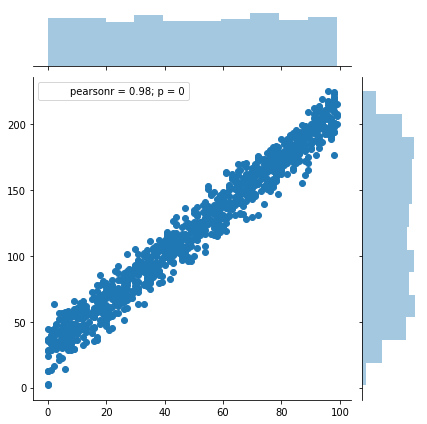X and y follow uniform distribution, but the error $\epsilon$ is generated from standard normal distribution with a boosting factor. Let us plot its histogram to verify the distribution

In :
sns.distplot(pop_error)

Out:
<matplotlib.axes._subplots.AxesSubplot at 0x1a171de8d0>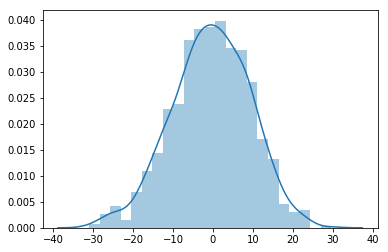### Predict using population¶¶

Let us predict the coefficients and intercept when using the whole dataset. We will compare this approach with CLT approach of breaking into multiple subsets and averaging the coefficients and intercepts

#### Using whole population¶¶

In :
from sklearn.linear_model import LinearRegression
X_train_full = rand_1kx.reshape(-1,1)
y_train_full = y.reshape(-1,1)

In :
y_train_full.shape

Out:
(1000, 1)
In :
lm.fit(X_train, y_train)

#print the linear model built
predicted_pop_slope = lm.coef_
predicted_pop_intercept = lm.intercept_

print("y = " + str(predicted_pop_slope) + "*X" + " + " + str(predicted_pop_intercept))

y = 1.795560991921382*X + 30.718916711669976


#### Prediction with 66% of data¶¶

In :
from sklearn.model_selection import train_test_split
X_train, X_test, y_train, y_test = train_test_split(rand_1kx, y, test_size=0.33)
print(X_train.size)

from sklearn.linear_model import LinearRegression
lm = LinearRegression()

670

In :
X_train = X_train.reshape(-1,1)
X_test = X_test.reshape(-1,1)
y_train = y_train.reshape(-1,1)
y_test = y_test.reshape(-1,1)

In :
y_train.shape

Out:
(670, 1)
In :
lm.fit(X_train, y_train)

#print the linear model built
predicted_subset_slope = lm.coef_
predicted_subset_intercept = lm.intercept_

print("y = " + str(predicted_subset_slope) + "*X"
+ " + " + str(predicted_subset_intercept))

y = 1.794887898705644*X + 29.857924099881075

##### Perform predictions and plot the charts¶¶
In :
y_predicted = lm.predict(X_test)
residuals = y_test - y_predicted


Fitted vs Actual scatter

In :
jax = sns.jointplot(y_test, y_predicted)
jax.set_axis_labels(xlabel='Y', ylabel='Predicted Y')

Out:
<seaborn.axisgrid.JointGrid at 0x1a179c9048>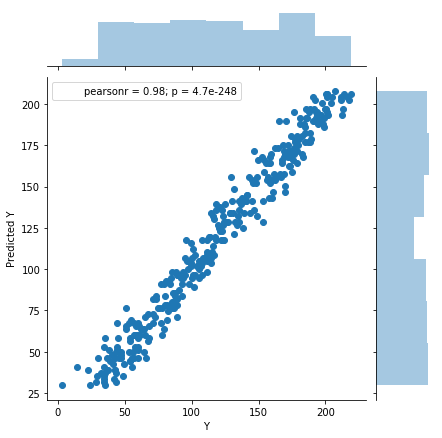In :
dax = sns.distplot(residuals)
dax.set_title('Distribution of residuals')

Out:
Text(0.5,1,'Distribution of residuals')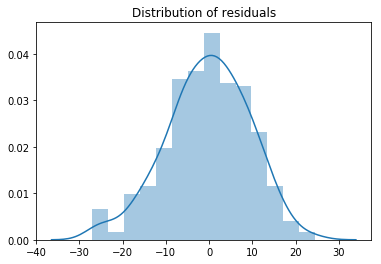In :
jax = sns.jointplot(y_predicted, residuals)
jax.set_axis_labels(xlabel='Predicted Y', ylabel='Residuals')

Out:
<seaborn.axisgrid.JointGrid at 0x1a1629f8d0>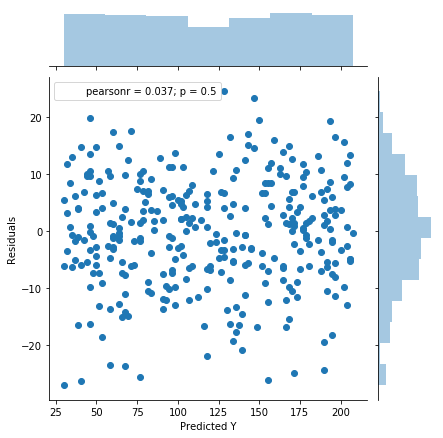In :
jax = sns.jointplot(y_test, residuals)
jax.set_axis_labels(xlabel='Y', ylabel='Residuals')

Out:
<seaborn.axisgrid.JointGrid at 0x1a16437eb8>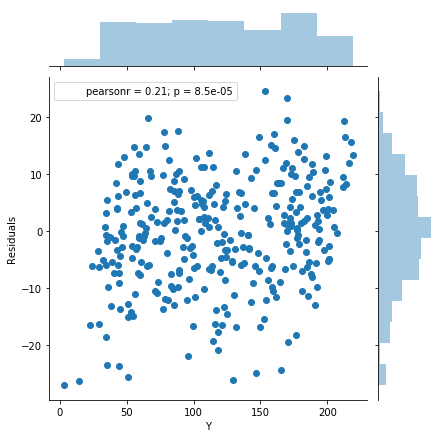### Predict using multiple samples¶¶

In :
pop_df = pd.DataFrame(data={'x':rand_1kx, 'y':y})

Out:
x y
0 38 85.149359
1 58 130.858406
2 15 67.280103
3 56 125.509595
4 19 55.793980
In :
pop_df.shape

Out:
(1000, 2)

#### Select 50 samples of size 200 and perform regression¶¶

In :
sample_slopes = []
sample_intercepts = []

for i in range(0,50):
# perform a choice on dataframe index
sample_index = np.random.choice(pop_df.index, size=50)

# select the subset using that index
sample_df = pop_df.iloc[sample_index]

# convert to numpy and reshape the matrix for lm.fit
sample_x = np.array(sample_df['x']).reshape(-1,1)
sample_y = np.array(sample_df['y']).reshape(-1,1)

lm.fit(X=sample_x, y=sample_y)

sample_slopes.append(lm.coef_)
sample_intercepts.append(lm.intercept_)


Plot the distribution of sample slopes and intercepts

In :
mean_sample_slope = np.mean(sample_slopes)
mean_sample_intercept = np.mean(sample_intercepts)

fig, ax = plt.subplots(1,2, figsize=(15,6))

# plot sample slopes
sns.distplot(sample_slopes, ax=ax)
ax.set_title('Distribution of sample slopes. Mean: '
+ str(round(mean_sample_slope, 2)))
ax.axvline(mean_sample_slope, color='black')

# plot sample slopes
sns.distplot(sample_intercepts, ax=ax)
ax.set_title('Distribution of sample intercepts. Mean: '
+ str(round(mean_sample_intercept,2)))
ax.axvline(mean_sample_intercept, color='black')

Out:
<matplotlib.lines.Line2D at 0x1a17ed97b8>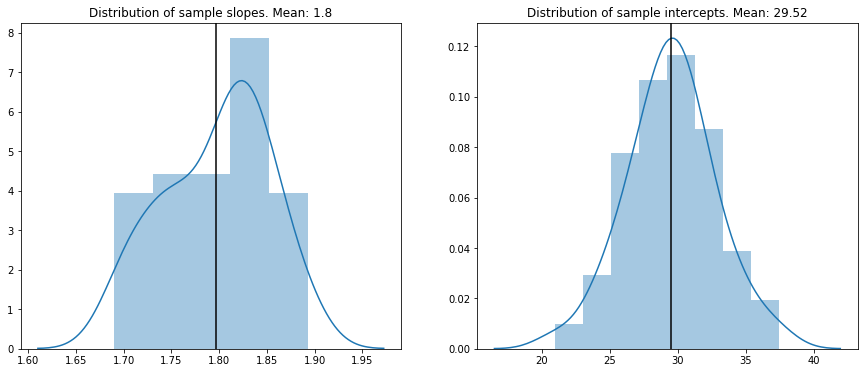### Conclusion¶¶

Here we compare the coefficients and intercepts obtained by different methods to see how CLT adds up.

In :
print("Predicting using population")
print("----------------------------")
print("Error in intercept: {}".format(pop_intercept - predicted_pop_intercept))
print("Error in slope: {}".format(pop_slope - predicted_pop_slope))

print("\n\nPredicting using subset")
print("----------------------------")
print("Error in intercept: {}".format(pop_intercept - predicted_subset_intercept))
print("Error in slope: {}".format(pop_slope - predicted_subset_slope))

print("\n\nPredicting using a number of smaller samples")
print("------------------------------------------------")
print("Error in intercept: {}".format(pop_intercept - mean_sample_intercept))
print("Error in slope: {}".format(pop_slope - mean_sample_slope))

Predicting using population
----------------------------
Error in intercept: -0.7189167116699764
Error in slope: 0.0044390080786180786

Predicting using subset
----------------------------
Error in intercept: 0.14207590011892535
Error in slope: 0.0051121012943560196

Predicting using a number of smaller samples
------------------------------------------------
Error in intercept: 0.4823977050074646
Error in slope: 0.002971759530004725


As we can see, error in quite small in all 3 cases, especially for slope. Prediction by averaging a number of smaller samples gives us much closer slope to population.

For intercept, the least error was with prediction using subset, which is still interesting as prediction using the whole population yielded poorer intercept!

In general, for really large datasets, that cannot be held in system memory, we can apply Central Limit Theorem for estimating slope and intercept by averaging over a number of smaller samples.